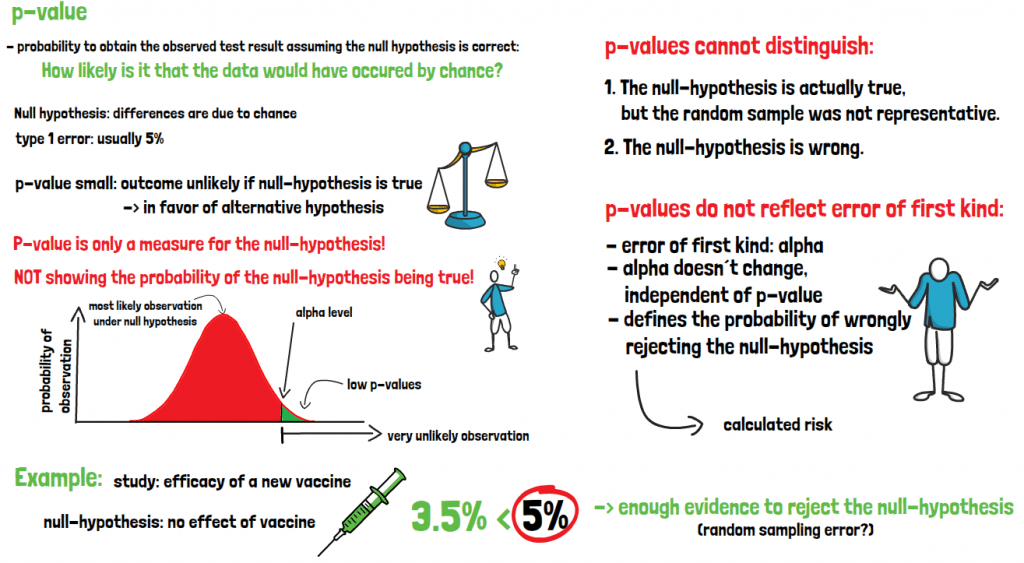# What is a p-value?P-values show how large the probability is to obtain the observed test results assuming the null hypothesis is correct. Or in other words, how likely it is that the data would have occurred by chance. The null hypothesis frequently states that there are no differences between groups for example, and that the differences or relationship found are plainly due to chance. In clinical studies usually an acceptable type one error of 5% is set as a threshold for the test decision. A small p-value indicates that it is very unlikely that the outcome would have been observed if the null hypothesis were true. In this case the decision made would be in favor of the alternative hypothesis, which would be more likely to explain the observed data. The p-value is only a measure for the null hypothesis, and not for the alternative hypothesis!

It is also notshowing the probability of the null hypothesis being true. It can only help in making conclusions about the probability of observing the data as it is, since the test is calculated assuming that the null-hypothesis is correct. A low p-value indicates that the data observed is very unlikely under the assumption that the null hypothesis is correct. It cannot distinguish if this outcome is due to the fact that

1. The null hypothesis is actually true, but the random sample was not representative or
2. The null hypothesis is wrong

The p-value does not, however, reflect the probability of committing an error of the first kind. The chance that your conclusion is wrong is defined only by alpha, which in any case still is 5%, independent of the p-value. This probability of being wrong is always given, as the random population sample could only by chance be in favor of the alternative hypothesis.

If you are interested in a visual presentation of this topic, please feel free to follow us on our GCP Mindset YouTube channel! If you would like to know more about how we could implement statistics in your clinical trial, send us a mail to statistics@gcp-service.com.